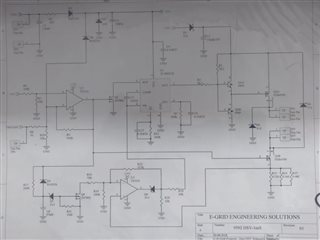# Low-side current sensing for high-performance cost-sensitive applications

Other Parts Discussed in Post: TLV906X

Applications that require the control of a motor typically involve some type of current-sensing circuitry. Being able to sense the current through the motor allows adjustments, such as speed, to the motor’s current state if needed.

For example, in drones, each of the motors that control the propellers typically use a low-side current-sensing circuit to steer, stabilize and lift the drone through the air. In power tools like drills and reciprocating saws, low-side current sensing controls the speed of the tool based on how hard users pull the trigger. These products typically require a cost-sensitive design because they are sold in the consumer market space. In this blog post, I’ll discuss how to design a low-side current-sensing circuit for cost-sensitive applications.

One cost-effective option when designing a low-side current-sensing circuit is to use an operational amplifier (op amp) in a noninverting configuration. Figure 1 is a schematic of a typical low-side current-sensing circuit using an op amp.Figure 1: Low-side current-sensing schematic

Equation 1 gives the transfer function of the circuit shown in Figure 1 as:where.

The design process for the low-side current-sensing circuit shown in Figure 1 breaks down into three simple steps:

1. Calculate the maximum shunt resistance. When current from the load (ILOAD) flows through the shunt resistor (RSHUNT), a voltage potential (VSHUNT) develops across RSHUNT. VSHUNT is seen as the “ground” for the system load. Therefore, I recommend keeping VSHUNT below 100mV at the maximum load current to avoid issues when interfacing with other systems that have a true 0V ground. Equation 2 calculates the maximum RSHUNTvalue as:2. Calculate the gain of the amplifier. The op amp amplifies VSHUNT in order to produce an output voltage swing of VOUT_MIN to VOUT_MAX, where VOUT_MIN and VOUT_MAXare the minimum and maximum output swing limits of the amplifier, respectively. Equation 3 calculates the gain of the amplifier to produce the desired output swing:Equation 4 calculates the size of the resistors, RF and RG, in the feedback network of the amplifier in order to set the gain calculated in Equation 3:3. Choose your amplifier. In low-side current-sensing applications, the common-mode voltage can be at or below ground if the current is bidirectional; therefore, the amplifier must have an input common-mode voltage range specified at or below ground. One device with an input common-mode voltage range that extends below ground is the TLV9062, a high-performance, general purpose amplifier designed for cost-sensitive applications.

The TLV906x high-performance general-purpose amplifier family is designed for cost-sensitive low-side current-sensing applications due to its gain bandwidth (10MHz), slew rate (6.5V/µs), offset voltage (0.3mV) and input common-mode voltage range, which is specified at 100mV below the negative supply voltage. Table 1 highlights a few of the TLV906x family’s typical specifications.

 Parameter Specification Supply voltage range ((V+)-(V-)) 1.8V to 5.5V Quiescent current 538µA Gain bandwidth product (GBP) 10MHz Input voltage noise 10nV/√Hz Slew rate 6.5V/µs Offset voltage 0.3mV Input bias current 0.5pA Input common-mode voltage (V-)-100mV to (V+)+100mV

The design in Figure 2 shows the final component values for a 0A to 0.5A low-side current-sensing circuit, with component values calculated by following steps 1 through 3.Figure 2: 0A to 0.5A low-side current-sensing schematic

Popular applications such as drones and power tools require cost-sensitive low-side current-sensing solutions for motor control. In this post, I simplified circuit design into three simple steps: determine the maximum shunt resistor, calculate the gain of the amplifier that produces the largest output swing and choose your amplifier. In my next post, I discuss how to properly lay out a printed circuit board (PCB) for a low-side current-sensing circuit.

• Hello Neet,

This sounds like an interesting application. Can you please post the question to our E2E Forums?

Thanks,

Pete

• Hello ,

We want to sense the current flowing through the Shunt Resistor but with a separate ground such that the current signal loop has a different voltage (36V) & different ground than the Measuring circuit with a 3.3V range. Kindly suggest how can we approach this use case, this is for cost-sensitive application.

• Hi Sethu Raman,

This would be a great question for our E2E forums. Can you please post your design question on TI's E2E forum

Thank you,

Tim Claycomb

• Hello Tim,

I need a circuit with following features,

1. Circuit input will be 24VDC pulse

2. With that I have to turn on a solenoid coil

3. Once current to the coil reaches some point, circuit should get off.

4. Currently I have a circuit(see attached).

5. Need suggestion to meet requirements.• Hi Sagar,

This circuit can be adjusted to accommodate just about any low side current sensing application by changing the resistor values to give the gain needed for the design.

Thank you,

Tim Claycomb